Volume 377, Issue 13, 17 May 2013, Pages 945–951

# Mass generation in the aether: Neutrino oscillations and massive gauge fields

• Department of Physics, Hiroshima University, 1-3-1 Kagami-yama, Higashi-Hiroshima 739-8526, Japan

## Abstract

Neutrino mixing is studied in an absolute spacetime conception based on a dispersive aether. The effect of the frequency-dependent permeability of the aether on the interference phase of neutrino mass eigenstates is analyzed. Neutrinos are treated as massless Dirac spinors, and mass eigenstates are due to the neutrino permeability of spacetime. The aether can also generate effective gauge masses, resulting in massive dispersion relations preserving the gauge symmetry. The propagators of gauge and spinor fields are derived, illustrating mass generation by isotropic permeability tensors in the aether frame, the rest frame of the cosmic background radiation.

#### Highlights

► Dirac neutrinos and gauge fields couple by permeability tensors to the aether. ► Effective neutrino and gauge masses are generated by permeability tensors. ► Oscillating flavor transition probabilities are due to effective mass-squares. ► The propagators of Dirac and gauge fields in a dispersive spacetime are derived. ► Gauge invariance is preserved by the effective mass in the propagators.

## Keywords

• Dispersive spacetime;
• Mass eigenstates of Dirac fermions;
• Neutrino mixing in a permeable spacetime;
• Effective neutrino mass and flavor oscillations;
• Effective mass-squares of gauge fields;
• Michelson–Morley experiments with neutrino beams

## 1. Introduction

We investigate the generation of mass in the dispersion relations of Dirac particles and gauge fields. Neutrino masses required for flavor oscillations as well as massive non-Abelian gauge fields are obtained by coupling the massless wave equations to permeability tensors. Flavor mixing , , ,  and  is compatible with sub- as well as superluminal neutrino velocities , ,  and , and arises in a dispersive spacetime due to the frequency-dependent permeabilities of the aether  and .

The aether defines a distinguished frame of reference, physically manifested as the rest frame of the isotropic cosmic microwave background radiation , , ,  and , and wave fields couple to the aether with isotropic permeability tensors in this frame , ,  and . We discuss the permeability tensors of Dirac fermions and gauge bosons, the interference phase of neutrino mass eigenstates ,  and , as well as gauge fixing and propagators in the dispersive aether, and potential Michelson–Morley experiments with neutrino beams.

Dirac particles freely propagating in the aether are described by the Dirac equation

equation1.1
γμgμνψ,ν+mψ=0coupled to a real symmetric permeability tensor gμν(ω), which depends on the frequency of the spinor modes ψ∝exp(ikμ(ω)xμ). The Dirac matrices satisfy γμγν+γνγμ=2ημν, where γ0 is anti-Hermitian, the γi are Hermitian, and the sign convention for the Minkowski metric is ημν=diag(−1,1,1,1). Latin indices run from one to three, Greek ones from zero to three; the latter are raised and lowered by the Minkowski metric. The wave 4-vector is kμ=(−ω,k), k=k(ω)k0, where k0 is the unit wave vector and k(ω) the wave number depending on the permeability tensor. In the aether frame, the permeability tensor is isotropic,
equation1.2where ε and μ are positive frequency-dependent permeabilities.

In Section 2, we discuss neutrino oscillations based on a massive Dirac equation (1.1) coupled by a permeability tensor (1.2) to the aether. In Section 3, we demonstrate that the interference phase can be generated by dispersive permeabilities (1.2) without a mass term in the wave equation. In Section 4, we study gauge and Proca fields in a permeable spacetime, in analogy to spinor fields. Each gauge component admits a permeability tensor which produces a massive dispersion relation without breaking the gauge invariance. In Section 5, we explain how permeability tensors generate effective mass in the propagators of massless gauge and Dirac fields. In Section 6, we study Dirac neutrinos in moving inertial frames coupled to an anisotropic permeability tensor and discuss Michelson–Morley neutrino experiments. In Section 7, we present our conclusions.

## 2. Neutrino mixing in a dispersive spacetime

equation2.1derived by squaring the Dirac equation, where j labels three mass eigenstates ψj, each characterized by permeabilities ε(j)(ω), μ(j)(ω), and a neutrino mass m(j); the k(j)(ω) are the respective wave numbers. Each eigenstate ψj satisfies a Dirac equation with mass m(j) and a permeability tensor defined by dispersive permeabilities ε(j)(ω) and μ(j)(ω). We use the shortcuts k(j)=k(j)(ωj), ε(j)=ε(j)(ωj) and μ(j)=μ(j)(ωj), where ωj is the frequency of the eigenstate plane wave ψj with wave 4-vector . The flavor transition amplitude reads 
equation2.2where l,l=e,μ,τ are the flavor indices of Dirac neutrinos and j is the eigenstate index. Ulj is a unitary matrix parametrized with Eulerian mixing angles and a CP violating phase. The Dj denote phase factors of the plane waves ψj,
equation2.3
Dj=exp(i(k(j)LωjT)),where the subscripts refer to emission and absorption. The neutrino wave vector is k(j)=k(j)k0, L is the path length between source and detector, and T the time of flight.

The transition probability |A(νlνl)|2 depends on the phase factors , defined by the phase difference

equation2.4δkij=k(i)(ωi)−k(j)(ωj).The increments
equation2.5Δεij(ω)=ε(i)(ω)−ε(j)(ω),Δμij(ω)=μ(i)(ω)−μ(j)(ω),as well as δωij in (2.4) are treated as small variations, and we expand δkij in linear order,
equation2.6The k(j)(ωj) are the eigenstate wave numbers (2.1), whose frequency derivative is the reciprocal group velocity, k(j)/∂ωj=1/υgr(j). We thus find
equation2.7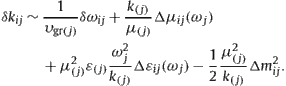In the phase difference δφij in (2.4), we put T=L/υgr(j) and substitute δkij,
equation2.8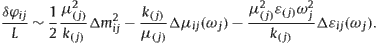If the time of flight T is defined by υgr(i) instead of υgr(j), this does not affect δφij in linear order, as these two velocities only differ by delta increments. For the same reason, we can drop the index j from ω. Similarly, if we replace the eigenstate index j by i in the three ratios on the right-hand side of (2.8), this does not affect the indicated linear order.

We split the eigenstate permeabilities and mass-squares in (2.1) as

equation2.9ε(j)(ω)=ε(ω)+δε(j)(ω),μ(j)(ω)=μ(ω)+δμ(j)(ω),where the increments , δε(j)(ω) and δμ(j)(ω) are small deviations from base values m2 (which can be zero) and ε(ω), μ(ω) (both close to 1, see after (3.3)). In the ratios of Eq. (2.8), we replace the permeabilities and the mass-squares in the wave numbers by their base values, since the expansion is linear in the delta increments. We thus arrive at the interference phase
equation2.10
where the wave number is independent of the eigenstate index.

## 3. Mass eigenstates generated by permeability tensors

If the mass eigenstates admit the same permeabilities, ε(j)(ω)=ε(ω), μ(j)(ω)=μ(ω), cf. (2.9), we can put Δμijεij=0 in the interference phase (2.10). Neglecting the mass-square m2 in the wave number k, cf. (2.9) and after (2.10), we find

equation3.1Alternatively, we may assume that the mass eigenstates ψj admit the same mass-square , so that . In this case, the interference phase (2.10) stems from the permeability increments (2.5). We put m2=0 in (2.9), so that k=μ(ω)ε(ω)ω and
equation3.2
δφij(ω)∼−(ε(ωμij+μ(ωεij)ωL,where Δμij(ω)=δμ(i)(ω)−δμ(j)(ω) and analogously Δεij(ω). The interference phase (3.1) is recovered from (3.2) by specifying the increments δε(j)(ω) and δμ(j)(ω) of the eigenstate permeabilities (2.9) as
equation3.3Here, a(ω) is an arbitrary real function that drops out in the phase difference (3.2); we may conveniently choose a=0,1, or a=μ/(ε+μ)≈1/2. Thus we have shown that the interference phase (3.1) is reproduced by the eigenstate permeabilities ε(j)(ω) and μ(j)(ω) defined in (2.9) and (3.3), without inserting mass terms m(j)ψj in the Dirac equations of the eigenstate plane waves. The refractive indices of the eigenstates ψj read, in leading order in , cf. (2.9) and (3.3),
equation3.4
and the wave numbers k(j)(ω)=nr(j)(ω)ω admit massive dispersion relations with an effective neutrino mass m(j) generated by the permeability increments (3.3).

A bound on the neutrino refractive index nr∼1/υgrk(ω)/ωμ(ω)ε(ω) at ω∼17 GeV is |1−nr|<3×10−6, based on recent measurements of the neutrino speed by the OPERA, BOREXINO, LVD and ICARUS Collaborations , ,  and . We use the nr(ω) estimate also for the factors μ(ω) and ε(ω). The neutrino flux from supernova SN1987A gives the bound |υgr−1|<2×10−9 at 10 MeV , so that we put μ(ω)≈ε(ω)≈1 in the low MeV region. An upper bound on the eigenstate masses m(j) from tritium β decay is 2 eV , assuming vacuum permeabilities in the eV range. The neutrino frequency ω is in the low MeV region (for reactor and solar νs) or low GeV region (accelerator, atmospheric νs). The path length L is of order 103 km and 104 km for accelerator and atmospheric νs. The differences of the eigenstate mass-squares are of order 10−4 eV2 or 10−3 eV2  and . To generate flavor oscillations, a phase difference |δφij|⩾1 is required for substantial interference in the squared amplitudes (2.2). As for the neutrino speed determined by the refractive index nr(j) in (3.4), we can ignore terms depending on the tiny (compared to O(10−6)) ratios , at least at length scales relevant for terrestrial experiments and solar νs. Accordingly, nrμ(ω)ε(ω) is independent of the eigenstate index, so that all neutrino flavors admit the same group velocity υgr=1/(ωnr(ω))∼1/nr.

## 4. Gauge and Proca fields in the aether: Massive dispersion relations preserving gauge invariance

The Lagrangian of a Proca field with negative mass-square reads

equation4.1where , , and are permeability tensors replacing the Minkowski metric ημν=diag(−1,1,1,1) in the vacuum Lagrangian  and . The signs in (4.1) are chosen in a way that is the negative mass-square of the tachyonic Proca field Aμ, and Fμν=Aν,μAμ,ν is the field tensor. A gauge-fixing term ∝1/ξ is included as Lagrange multiplier. Massive Proca fields are obtained by replacing . The 3D field strengths are Ei=Fi0, Bk=εkijFij/2, and inversely Fij=εijkBk. Thus, Ei=A0,iAi,0, Bk=εkijAj,i, where εijk is the totally antisymmetric tensor. The field is coupled to a current jμ=(−ρ,j). The permeability tensors and are real and frequency dependent. The are symmetric, and is antisymmetric in the first as well as second index pair, and symmetric regarding the interchange αβμν of the index pairs. We define the inductive potential, field tensor and current as
equation4.2and write , so that jΩ0=−ρΩ. Greek indices are raised and lowered with the Minkowski metric. The Lagrangian (4.1) can thus be written as The 3D inductions are Di=H0i, Hi=εimnHmn/2, and inversely Hmn=Hjεjmn, so that FαβHαβ=2(BHED). The constitutive equations relating the 3D inductions to the field strengths read
equation4.4where the permeabilities εij(ω) and μij(ω) are real symmetric 3-tensors, and is a mixed tensor. We need not assume different tensors in the two relations (4.4), as the Lagrangian would only depend on their sum via FαβHαβ. The permeability tensor in (4.1) is defined by these 3-tensors,
equation4.5All other components of follow from the mentioned symmetries or vanish. Inversely,
equation4.6In the isotropic aether frame, we can put , cf. after (4.11), but in moving frames the tensor does not vanish, cf. after (5.4).

Euler variation of Lagrangian (4.3) gives the field equations and gauge condition

equation4.7equation4.8For instance, the Lorentz condition follows from current conservation if the mass-square does not vanish and the gauge-fixing term in the Lagrangian is dropped, ξ=∞. Eqs. (4.7) are equivalent to
equation4.9where jΩ0=−ρΩ, and the constitutive relations (4.4) apply. In the massless case , the gauge-invariant Maxwell equations in a permeable spacetime are recovered by imposing the Lorentz condition on the vector potential, also see (4.11).

We consider isotropic permeability tensors, cf. (4.2), (4.4) and (4.5),

equation4.10with permeabilities (ε,μ), (ε0,μ0) and (Ω0,Ω) depending on the frequency ω of the wave modes in the aether frame . The tensor couples the current to the wave modes, cf. (4.1) and (4.2), and amounts to a varying coupling constant if Ω0(ω) coincides with 1/Ω(ω). We note Hk=εkijAj,i/μ and Dk=ε(A0,kAk,0), cf. after (4.1) and (4.4). The isotropic field equations for the vector potential read, cf. (4.9),
equation4.11where . The isotropic tensor in the constitutive relations (4.4) drops out in the field equations, since the κEB term in the Lagrangian (see after (4.3)) is a divergence, 2EkBk=(εαβμνAβAμ,ν),α. In  and , we studied the field Eqs. (4.11) in the limit ξ=∞. In the following, we consider the opposite limit, with finite ξ. That is, we drop the mass term in Lagrangian (4.1) and generate a positive or negative mass-square in the dispersion relation by way of the permeability tensors (4.10).

The isotropic field equations (4.11) (with ) admit the plane-wave solutions

equation4.12where we have split the field into a transversal and longitudinal component, , with wave number and , respectively. The gauge-fixing constant ξ does not explicitly show in (4.12). If the gauge-fixing term is dropped by putting ξ=∞, the general plane-wave solution of frequency ω is still (4.12), but with kL(ω) unspecified. A finite ξ gives a well-defined wave number to the longitudinal component , which is a gauge transformation, , .

The permeability tensor defines the gauge-fixing term in Lagrangian (4.3). We identify ε0=ε and μ0=μ, so that in (4.10) reads , , . The transversal and longitudinal components of the vector potential (4.12) thus admit the same wave number, , where ε(ω) and μ(ω) are the permeabilities defining , cf. (4.5) and (4.10). A massive dispersion relation k2=ω2m2 is induced by permeabilities satisfying εμ=1−m2/ω2, without a mass term in the Lagrangian, and longitudinal field components remain gauge transformations. If we impose the Lorentz condition , the gauge-fixing term drops out in the field equations (4.11) (with ), and they become gauge invariant despite of the massive dispersion relation induced by the permeabilities. [A negative mass-square in the dispersion relation requires permeabilities related by . A tachyonic dispersion relation preserving the hermiticity of the Dirac Hamiltonian is obtained by replacing in (5.14).] As for electromagnetic fields, we use vacuum permeabilities, ε0=ε=1 and μ0=μ=1, defining the constant speed in the Lorentz boosts, so that the electromagnetic Michelson–Morley isotropy is preserved in moving frames.

## 5. Effective mass-squares in propagators of dispersive gauge and Dirac fields

The foregoing admits generalization to non-Abelian gauge fields , each component being coupled to the aether by permeabilities ε(α)(ω) and μ(α)(ω), α=1,…,N. We consider the Lagrangian, cf. (4.3),

equation5.1with field tensor , inductions , , and inductive current . We write the gauge index α of the permeability tensors , and in parentheses, to indicate that it is not a summation index unless in a product where α appears twice without parentheses. When calculating the propagator, it suffices to use the quadratic component of the Lagrangian and to put the coupling constant g in the field tensor to zero, so that the linearized field equations are independent of the structure constants , , cf. (4.7). The tensors and are isotropic in the aether frame, cf. (4.8) and (4.10) and after (4.12): The isotropic permeability tensor reads, cf. (4.5) and (4.10),
equation5.3with all other components following from symmetry properties, cf. after (4.1). Without loss of generality, we can put κ(α)(ω)=0, cf. after (4.11), and reduce to a symmetric tensor :
equation5.4The refractive indices give rise to massive dispersion relations k(α)=ωnr(α) with an effective gauge mass m(α) for each gauge component , cf. (4.12). The tensors transform contravariantly under Lorentz boosts, cf.  and Section 6.

We substitute the plane waves and into the linearized isotropic Lagrangian (5.1),

equation5.5and perform a time average over a period to find
equation5.6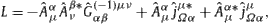where denotes the Gaussian kernel
equation5.7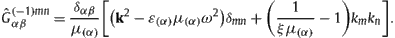Euler variation of L with respect to gives . The inverse kernel is the propagator ,
equation5.8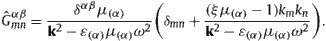At ξ=0, this propagator satisfies the Lorentz condition , where kμ=(−ω,k). A frequency-dependent gauge parameter is also admissible, which can even be different for each gauge component α; for instance, we may substitute ξξ(α)=1/μ(α), which diagonalizes the propagator. Observable gauge-invariant quantities such as the linear inductions and obtained from the solution are independent of the gauge parameter ξ, and the same holds true for the field strengths and :
equation5.9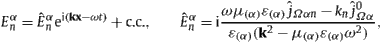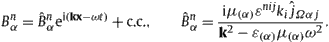A more compact representation of kernel (5.7) and propagator (5.8) is

equation5.10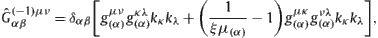equation5.11where denotes the inverse of the diagonal matrix , cf. (5.2) and (5.4). We can replace in the gauge-fixing term of Lagrangian (5.1), so that the inductive field is . This amounts to a conformal rescaling ξξ/μ(α) of the gauge parameter in propagator (5.11), which becomes manifestly covariant in this gauge, , cf. (4.8) and after (5.8).

We specify the permeabilities as , with positive infinitesimal constants regularizing the denominators of in (5.8) as . The wave numbers thus admit an infinitesimal imaginary part and a massive dispersion relation , where is the diverging attenuation length of the field component α in the infinitesimally dissipative aether . To find the lifetime associated with this attenuation length, we put ω=E−iΓ(α)(E)/2, and determine the decay rate Γ(α) by solving , obtaining in linear order in the infinitesimal . In this way, one can switch between complex space and time components of the plane-wave phase, using either k(α)k0xωt or (where we may again write ω for E). Thus the squared wave function scales with exp(−k0x/δ(α)) or . The lifetime of a mode in the aether is related to its attenuation length by δ(α)υgr(α)/Γ(α), where is the group velocity. At , the permeabilities are real and symmetric on the real axis, satisfying and in the complex plane. The antipropagator is found by variation of L in (5.6).

To obtain the Dirac propagator, we start with the Lagrangian of a spinor multiplet ψj,

equation5.12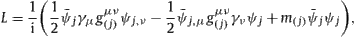where is the adjoint spinor, and the permeability tensor with components , , , see after (2.1). The Dirac equation reads , where j is a multi-index labeling flavor, color, etc. We write j in parentheses if it is not meant as a summation index, cf. after (5.1). On substituting plane waves , we find , with kernel . To invert , we note γμγν=ημν+σμν, where σμν is antisymmetric, so that the product of is , with the squared permeability tensor . Hence,
equation5.13where we used the isotropic permeability tensor stated after (5.12). We note that , cf. (2.1).

We drop all mass terms in (5.12) and (5.13), that is we use the massless Dirac equation, cf. after (5.12), and specify the refractive indices nr(j)=ε(j)μ(j) of the multiplet ψj as, cf. (3.4),

equation5.14
We also put μ(j)=μ(ω) and assume ε(ω) and μ(ω) to be close to one, infinitesimal , as well as m(j)/ω≪1, so that the permeability tensor (stated after (5.12)) is close to the Minkowski metric ημν. We thus find the propagator of the massless Dirac equation as, cf. (5.13),
equation5.15In the nominator of (5.15), we can put in nr(j). The are positive infinitesimal constants defining the Green function (here the propagator), i.e. the integration path around its pole; the complex wave numbers k(j)=ωnr(j) result in infinitesimally damped wave fields, cf. before (5.12).

In propagator of the massive Dirac equation, cf. (5.13), we put ε(j)=ε(ω),μ(j)=μ(ω), and use the integration path prescription as in (5.15). Since the neutrino mass is much smaller than the neutrino energy, cf. the end of Section 3, we can drop the iμ(j)m(j)/ω term in (5.13) and expand in (5.14), so that the propagators (5.13) and (5.15) coincide in leading order in an m(j)/ω expansion. The mass-square determining the pole singularity of is recovered in propagator without invoking a mass term in the Dirac Lagrangian (5.12).

## 6. Dirac equation and permeability tensor in moving inertial frames: Michelson–Morley experiments with neutrino beams

We consider an inertial frame uniformly moving in the aether with velocity υr=υrυr,0. Unit vectors are denoted by a zero subscript. The spacetime coordinates in the rest frame of the aether (aether frame) are denoted by xμ=(t,x) and in the inertial frame by yμ=(τ,y). The proper orthochronous Lorentz boost relating the frames is

equation6.1The inverse transformation is found by changing the sign of the relative velocity υr,0. Contravariant quantities transform from the aether frame to a moving inertial frame like, covariant ones like , and mixed quantities according to this pattern. Indices are raised and lowered with the invariant Minkowski metric ημν=diag(−1,1,1,1). Energy and momentum variables are denoted by kμ=(−ω,k) in the aether frame and by pμ=(−E,p) in the inertial frame, and .

In the aether frame, the Dirac equation (1.1) reads

equation6.2coupled to the isotropic permeability tensor in (1.2). We consider plane waves , where is a constant 4-spinor. The wave vector kμ=(−ω,k), k=k(ω)k0, satisfies the dispersion relation (2.1), k2=μ2(ε2ω2m2).

In the moving inertial frame related by the Lorentz boost (6.1) to the aether frame, the permeability tensor is anisotropic, . The tensors are independent of space and time coordinates; they only depend on the frequency ω of the wave modes in the aether frame, which is the universal frame of reference. We denote the boosts (6.1) by x=Λ(y) and y=Λ−1(x). To find the Dirac equation in the inertial frame, we write Eq. (6.2) as

equation6.3and consider a spinor field ψin(ω;y) in the inertial frame, related to a plane-wave solution ψae(ω;x) of Eq. (6.2) by a similarity SΛ, so that ψin(ω;y)=SΛψae(ω;Λ(y)) and . We may thus write Eq. (6.3) as
equation6.4By choosing the matrix SΛ as a solution of ,
equation6.5where γr denotes the Lorentz factor, cf. (6.1), we find the Dirac equation in the inertial frame,
equation6.6The inverse similarity is obtained by changing the sign of the velocity unit vector in (6.5), so that . The Dirac matrices in (6.5) satisfy γμγν+γνγμ=2ημν, where γ0 is anti-Hermitian and the γn and SΛ are Hermitian, and . A plane-wave solution of (6.2) in the aether frame is transformed into solving (6.6), where . The dispersion relations in the aether frame and the inertial frame can be written as and , respectively, where , cf. (5.13). The Dirac equation in (6.2) and (6.6) has a manifestly covariant appearance as well, but is not covariant at all, since the anisotropic permeability tensor of the inertial frame depends on the energy ω of the wave field in the aether frame, which is the universal frame of reference; is obtained by applying a Lorentz boost to the isotropic reference tensor of the aether frame.

As for Michelson–Morley experiments, we consider neutrinos propagating with constant (group) velocity υgr=υgrυgr,0 in the aether frame where the permeability tensor is isotropic. An inertial laboratory frame moves with constant relative speed υr=υrυr,0 in the aether. The frames are related by the Lorentz boost (6.1). In the context of neutrino velocity experiments , ,  and , the inertial frame is the neutrino baseline frame (rest frame of neutrino source and detector), slowly moving with a relative velocity of order υr=O(10−3) in the aether, cf. the discussion after (6.10). In the moving inertial frame, the neutrino velocity is denoted by υin=υinυin,0 and related to the group velocity in the aether frame by the relativistic addition law (linearized in the relative speed υr),

equation6.7and inversely,
equation6.8cosθgrcosθin+υrυinsin2θin,where cosθgr=υgr,0υr,0 and cosθin=υin,0υr,0, which applies in slowly moving inertial frames, υr1. The velocities υgr and υin are either both sub- or superluminal, which can be seen from the exact velocity addition law . The transformation (6.7) and its inverse (6.8) are complemented by the Doppler shift connecting the neutrino energy E measured in the inertial frame to the energy ω of the wave mode in the aether frame according to pμ=kαΛαμ, cf. after (6.1). The Doppler shift (linearized in υr) and its inverse read
equation6.9
Eω(1nr(ω)υrcosθgr),ωE(1+nr(E)υrcosθin),where nr(ω) is the refractive index
equation6.10
nr(ω)=k(ω)ω=με1m2ε2ω2,1υgr=(ωnr(ω)),defined by the dispersion relation in the aether frame, cf. after (6.2). nr(E) in (6.9) means nr(ω) taken at ω=E. (Terms quadratic in υr are neglected in (6.9).) The Doppler shift from the aether frame to a moving inertial frame depends not only on the relative velocity but also on the refractive index, which is a function of the neutrino frequency ω in the aether frame. Due to the dispersive permeabilities, the neutrino group velocity υgr(ω) is energy dependent even for massless neutrinos.

The group velocity υgr in the aether frame is obtained via transformation (6.8), once we have measured the neutrino velocity υin in the inertial laboratory frame and the angle cosθin between inertial velocity and relative speed. Assuming adiabatic frequency variation of the refractive index, ωnr(ω)/nr1, we can identify nr1/υgr, cf. (6.10). The neutrino frequency ω in the aether frame is then found by measuring the neutrino energy E in the inertial frame, ω/E1+(υr/υgr)cosθin, cf. (6.9). Adiabatic variation of nr(ω) requires adiabatic permeabilities, ωε(ω)/ε1 and ωμ(ω)/μ1, as well as a small neutrino mass, m/ω1, cf. (6.10).

The neutrino velocity υin in the laboratory frame depends on the angle cosθin between the neutrino velocity and the relative velocity of the inertial frame in the aether, cf. (6.7). The minimal (maximal) neutrino velocity υin is attained at θin=0 or θin=π, when the velocity vectors are aligned or opposite, depending on whether the refractive index nr1/υgr is larger or smaller than one (sub- or superluminal group velocity in the aether frame). The maximal variation of the inertial neutrino speed in the baseline frame is thus δυin2υr(1/n2r1), cf. (6.7). If the neutrino beam can arbitrarily be rotated and the neutrino velocity υin is measured as a function of the angles parametrizing the rotation, one can in this way determine the relative speed υr of the inertial frame (here the local baseline rest frame of source and detector) in the aether, as attempted in the optical Michelson–Morley experiment. At θin=π/2, when the velocities υr and υin are orthogonal, the measured inertial neutrino speed υin coincides with the neutrino group velocity υgr1/nr in the aether frame (that is, the linear order in the relative speed υr vanishes, cf. (6.8)). The OPERA Collaboration derived the bound υin−1=(2.7±6.5)×10−6 on the neutrino velocity . To illustrate the angular dependence of this velocity, we use υin−1≈2.7×10−6 and the relative speed υr≈1.2×10−3 of the Solar system barycenter in the cosmic microwave background. (Earthʼs relative velocities in the Solar system are by at least one order smaller.) We note υgr−1=(υin−1)(1+O(υr)), cf. (6.8), and find a maximal angular variation of δυin≈4υr(υin−1)≈1.3×10−8 for the neutrino velocity in the inertial frame, which is still by three orders smaller than the accuracy of current υin measurements , ,  and . The isotropic aether frame is identified as the rest frame of the microwave background defined by vanishing temperature dipole anisotropy  and .

## 7. Conclusion

We demonstrated that effective mass-squares can be generated by permeability tensors in spinor as well as gauge fields, for fermions and bosons alike, familiar from the electrodynamics of dispersive dielectric media. This analogy is evident in each step, from the Lagrangians to the propagators, cf. Sections 4 and 5. Massless gauge fields coupled to the aether by dispersive permeability tensors admit massive dispersion relations preserving the gauge invariance. Mass generation by a permeability tensor is particularly attractive in the case of neutrino mixing, when small masses in the sub-eV range are needed  and , since such masses are generated by permeability tensors close to the Minkowski metric, cf. Sections 2 and 5. Interference factors in the flavor transition probabilities are due to phase differences of the mass-eigenstate plane waves, which result in oscillations if the neutrino path length is sufficiently large. All neutrino flavors propagate at the same group velocity, υgr≈1/(εμ), as the refractive indices of high-energy mass eigenstates only differ by negligible terms of order , cf. Section 3. Sub- and superluminal neutrino velocities close to the speed of light can be described on equal footing by permeability tensors; the permeabilities defining the dispersion relations are slightly larger than one in the case of subluminal neutrino speeds and smaller than one for superluminal neutrinos. The neutrino velocity measured in the baseline frame (rest frame of neutrino source and detector) depends on the angle between the neutrino beam (baseline) and its relative velocity in the aether. This angular dependence can be used to locally determine the velocity of source and detector in the aether, cf. Section 6, without invoking the temperature anisotropy of the cosmic microwave background in the neutrino baseline frame.

## References

• 
• Phys. Rev. Lett., 108 (2012), p. 171803